# Simulating diffusion networks: Using the `rdiffnet` function

In this example we compare 3 different simulations that use the same baseline (seed) network, a scale-free generated via `rgraph_ba` (Barabasi-Albert) with parameter `m=4` (number of new ties that each added node includes in the graph). The only difference between the three simulations is that we use a different set of seed adopters, “random”, “central” and “marginal”. All three cases start with 5% of the network having adopted the innovation.

``````library(netdiffuseR)
s <- 11532
set.seed(s)
diffnet_ran <- rdiffnet(200, 20, "random", seed.p.adopt = .1,
seed.graph = "small-world",
rgraph.args = list(undirected=FALSE, k=4, p=.5),
threshold.dist = function(x) 0.3)``````
``````## Warning in (function (graph, p, algorithm = "endpoints", both.ends = FALSE, :
## The option -copy.first- is set to TRUE. In this case, the first graph will be
## treated as a baseline, and thus, networks after T=1 will be replaced with T-1.``````
``````set.seed(s)
diffnet_cen <- rdiffnet(200, 20, "central", seed.p.adopt = .1,
seed.graph = "small-world",
rgraph.args = list(undirected=FALSE, k=4, p=.5),
threshold.dist = function(x) 0.3)``````
``````## Warning in (function (graph, p, algorithm = "endpoints", both.ends = FALSE, :
## The option -copy.first- is set to TRUE. In this case, the first graph will be
## treated as a baseline, and thus, networks after T=1 will be replaced with T-1.``````
``````set.seed(s)
diffnet_mar <- rdiffnet(200, 20, "marginal", seed.p.adopt = .1,
seed.graph = "small-world",
rgraph.args = list(undirected=FALSE, k=4, p=.5),
threshold.dist = function(x) 0.3)``````
``````## Warning in (function (graph, p, algorithm = "endpoints", both.ends = FALSE, :
## The option -copy.first- is set to TRUE. In this case, the first graph will be
## treated as a baseline, and thus, networks after T=1 will be replaced with T-1.``````

Furthermore, we can take a more detail view of what’s going on in each network using the `summary` method. For example, lets take a look at the marginal network:

``summary(diffnet_mar)``
``````## Diffusion network summary statistics
## Name     : A diffusion network
## Behavior : Random contagion
## -----------------------------------------------------------------------------
##  Period   Adopters   Cum Adopt. (%)   Hazard Rate   Density   Moran's I (sd)
## -------- ---------- ---------------- ------------- --------- ----------------
##        1         20        20 (0.10)             -      0.02 -0.01 (0.00)
##        2          2        22 (0.11)          0.01      0.02 -0.00 (0.00)
##        3          2        24 (0.12)          0.01      0.02 -0.00 (0.00)
##        4          1        25 (0.12)          0.01      0.02 -0.00 (0.00)
##        5          1        26 (0.13)          0.01      0.02 -0.00 (0.00) *
##        6          4        30 (0.15)          0.02      0.02  0.00 (0.00) ***
##        7          1        31 (0.15)          0.01      0.02  0.00 (0.00) ***
##        8          5        36 (0.18)          0.03      0.02  0.00 (0.00) ***
##        9          4        40 (0.20)          0.02      0.02  0.01 (0.00) ***
##       10          5        45 (0.23)          0.03      0.02  0.01 (0.00) ***
##       11         13        58 (0.29)          0.08      0.02  0.01 (0.00) ***
##       12         15        73 (0.36)          0.11      0.02  0.02 (0.00) ***
##       13         21        94 (0.47)          0.17      0.02  0.02 (0.00) ***
##       14         36       130 (0.65)          0.34      0.02  0.01 (0.00) ***
##       15         46       176 (0.88)          0.66      0.02  0.00 (0.00) ***
##       16         23       199 (0.99)          0.96      0.02 -0.01 (0.00)
##       17          1       200 (1.00)          1.00      0.02               -
##       18          0       200 (1.00)          0.00      0.02               -
##       19          0       200 (1.00)          0.00      0.02               -
##       20          0       200 (1.00)          0.00      0.02               -
## -----------------------------------------------------------------------------
##  Left censoring  : 0.10 (20)
##  Right centoring : 0.00 (0)
##  # of nodes      : 200
##
##  Moran's I was computed on contemporaneous autocorrelation using 1/geodesic
##  values. Significane levels  *** <= .01, ** <= .05, * <= .1.``````

At a first look, printing the networks, we can see that they differ in the number of adopters, as the adoption rate shows:

``diffnet_ran; diffnet_cen; diffnet_mar``
``````## Dynamic network of class -diffnet-
##  Name               : A diffusion network
##  Behavior           : Random contagion
##  # of nodes         : 200 (1, 2, 3, 4, 5, 6, 7, 8, ...)
##  # of time periods  : 20 (1 - 20)
##  Type               : directed
##  Final prevalence   : 1.00
##  Static attributes  : real_threshold (1)
##  Dynamic attributes : -``````
``````## Dynamic network of class -diffnet-
##  Name               : A diffusion network
##  Behavior           : Random contagion
##  # of nodes         : 200 (1, 2, 3, 4, 5, 6, 7, 8, ...)
##  # of time periods  : 20 (1 - 20)
##  Type               : directed
##  Final prevalence   : 1.00
##  Static attributes  : real_threshold (1)
##  Dynamic attributes : -``````
``````## Dynamic network of class -diffnet-
##  Name               : A diffusion network
##  Behavior           : Random contagion
##  # of nodes         : 200 (1, 2, 3, 4, 5, 6, 7, 8, ...)
##  # of time periods  : 20 (1 - 20)
##  Type               : directed
##  Final prevalence   : 1.00
##  Static attributes  : real_threshold (1)
##  Dynamic attributes : -``````

So, as expected, the network that used central nodes as first adopters is the one that reached the highest adoption rate, 0.95; meanwhile the network that used marginal nodes as seed has the lowest adoption rate, 0.56. Lets compare the set of initial adopters graphically

``````cols <- c("lightblue","green", "blue")

par(mfcol=c(1,3), mai = c(0, 0, 1, 0), mar = rep(1, 4) +  0.1)
set.seed(s);plot(diffnet_ran, main="Random seed")``````
``````## Warning in sprintf(main, x\$meta\$pers[t]): one argument not used by format
## 'Random seed'``````
``set.seed(s);plot(diffnet_cen, main="Central seed")``
``````## Warning in sprintf(main, x\$meta\$pers[t]): one argument not used by format
## 'Central seed'``````
``coords <- set.seed(s);plot(diffnet_mar, main="Marginal seed")``
``````## Warning in sprintf(main, x\$meta\$pers[t]): one argument not used by format
## 'Marginal seed'``````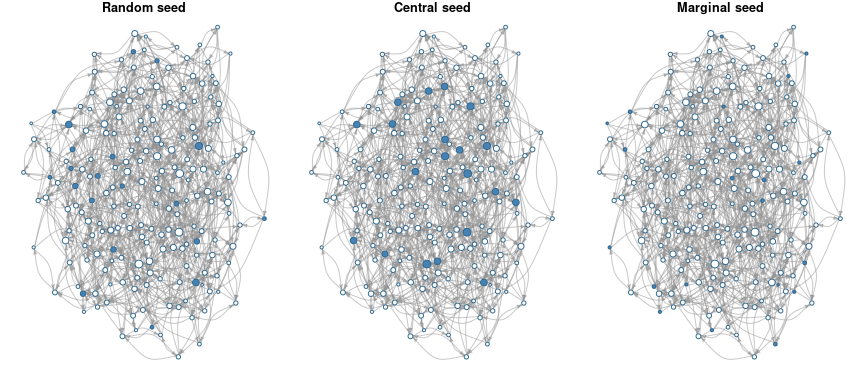``par(oldpar)``

An interesting way of visualizing the diffusion process is using the `plot_diffnet` function. In this case, instead of plotting all the 20 periods (networks), we only focus on a subset (henceforth we use the `slices` argument).

``plot_diffnet(diffnet_ran, slices = c(1,4,8,12,16,20), layout=coords)``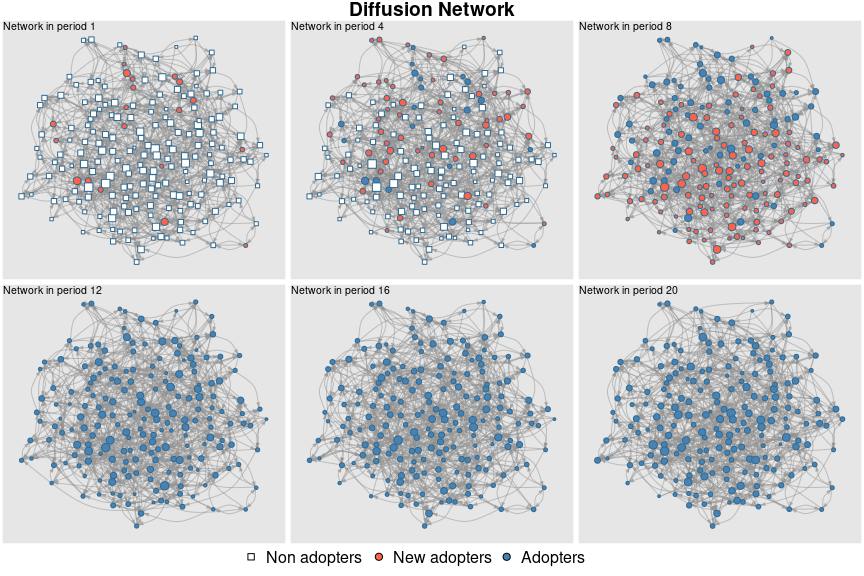## Diffusion process

An easy way to compare these three networks is by checking the cumulative adoption counts, in particular, the proportion. Using the function `plot_adopters` we can achieve our goal

``````plot_adopters(diffnet_ran, bg = cols, include.legend = FALSE, what="cumadopt")

legend("topleft", bty="n",
legend = c("Random","Central", "Marginal"),
fill=cols)``````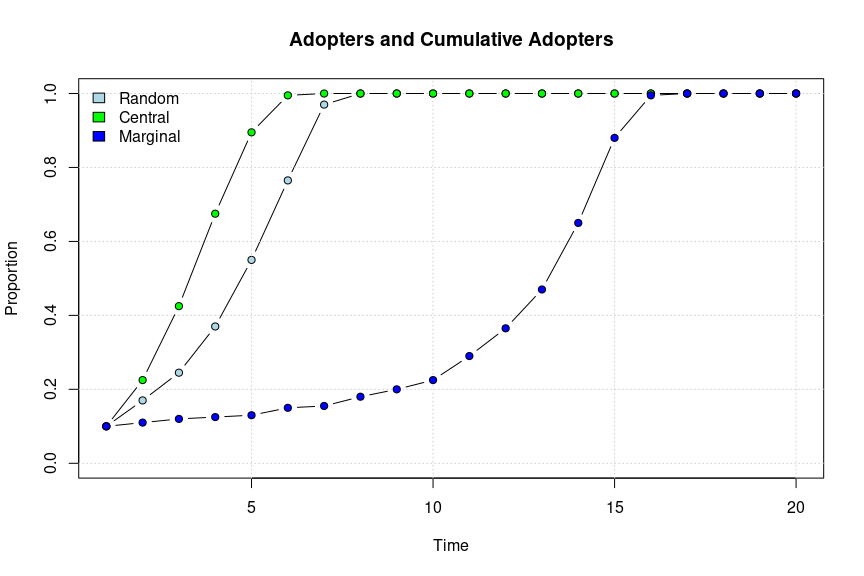Comparing hazard rates we can do the following

``````plot_hazard(diffnet_ran, ylim=c(0,1), bg=cols)

legend("topleft", bty="n",
legend = c("Random","Central", "Marginal"),
fill=cols)``````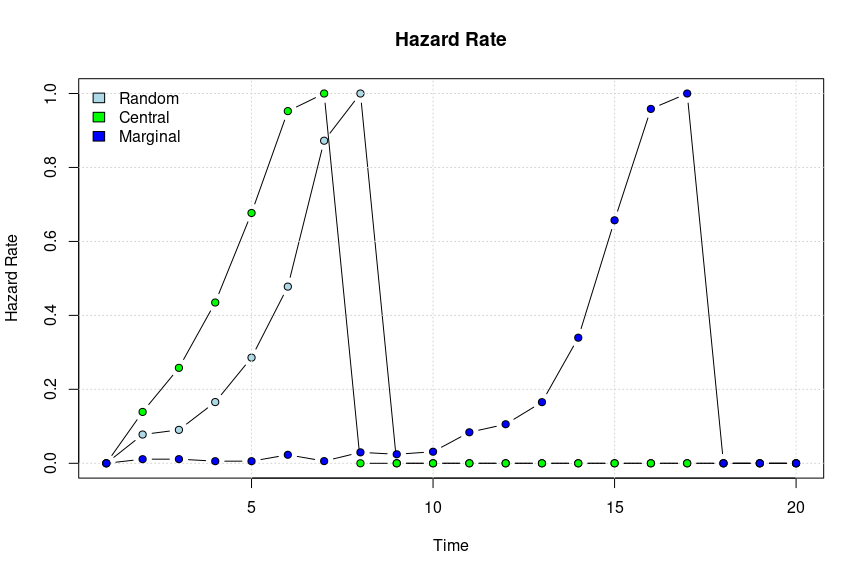Furthermore, we can calculate infectiousness and susceptibility on each network and see whether both are correlated in each one of the processess.

``````plot_infectsuscep(diffnet_ran, bins=15, K=3,
main = "Distribution of Infectiousness and\nSusceptibility (Random)")``````
``````## Warning in plot_infectsuscep.list(graph\$graph, graph\$toa, t0, normalize, : When
## applying logscale some observations are missing.``````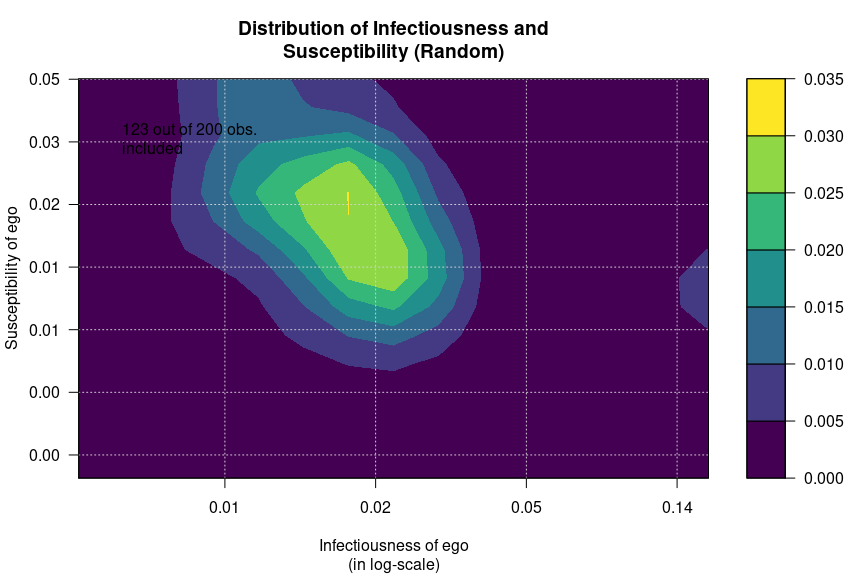``````plot_infectsuscep(diffnet_cen, bins=15, K=3,
main = "Distribution of Infectiousness and\nSusceptibility (Central)")``````
``````## Warning in plot_infectsuscep.list(graph\$graph, graph\$toa, t0, normalize, : When
## applying logscale some observations are missing.``````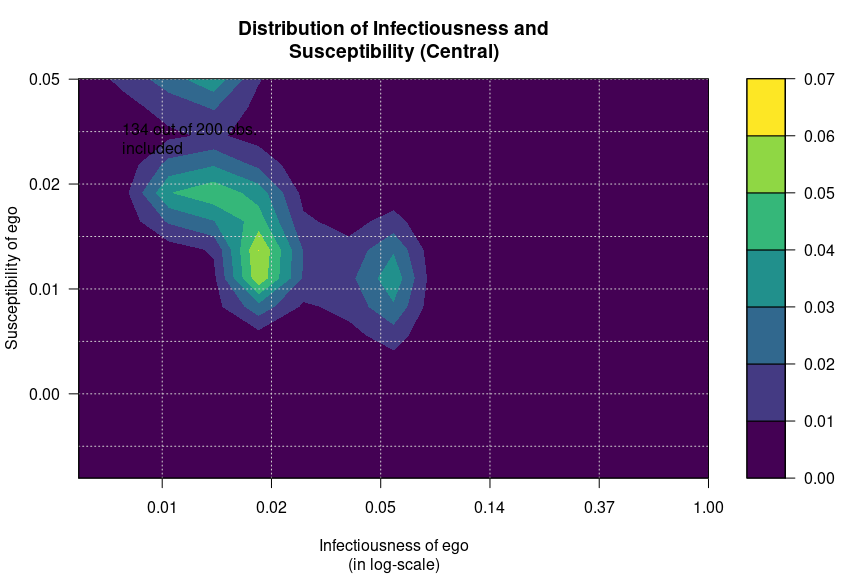``````plot_infectsuscep(diffnet_mar, bins=15, K=3,
main = "Distribution of Infectiousness and\nSusceptibility (Marginal)")``````
``````## Warning in plot_infectsuscep.list(graph\$graph, graph\$toa, t0, normalize, : When
## applying logscale some observations are missing.``````